# Test: Electronic Devices - 1

## 15 Questions MCQ Test GATE ECE (Electronics) 2023 Mock Test Series | Test: Electronic Devices - 1

Description
Attempt Test: Electronic Devices - 1 | 15 questions in 45 minutes | Mock test for Electronics and Communication Engineering (ECE) preparation | Free important questions MCQ to study GATE ECE (Electronics) 2023 Mock Test Series for Electronics and Communication Engineering (ECE) Exam | Download free PDF with solutions
QUESTION: 1

### A BJT with one junction forward biased and either Emitter or collector is open operates in

Solution:

Charge storage will be positive and higher so it must enter into saturation.

QUESTION: 2

### A, N-channel Si (εr = 12) FET with a channel width a = 2 × 10−6 meter is doped with 1021 e’s / m3. The value of pinch-off voltage is _________ V.

Solution: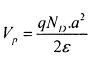QUESTION: 3

### A diode which is in reverse bias at 3 volt has a junction capacitance of 20 pF when reverse bias is increased to 24 volt then junction capacitance becomes 8 pF. The doping profile and contact potential are

Solution: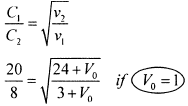QUESTION: 4

A potential difference of 5 volt is applied across a uniform wire of length 50 meter. Calculate drift velocity of electrons through the wire, If relaxation time is 10−14 sec. (Given Mass of electron, m = 9.1 × 10−31 kg)

Solution: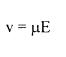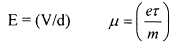QUESTION: 5

For an ideal Ge forward bias diode, the change in diode voltage to increase forward bias diode current 10 times is _________ mV.

Solution: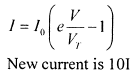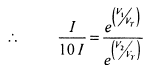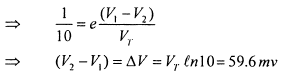QUESTION: 6

Which one of the following statements is correct?In the context of I.C. fabrication metallization means

Solution:

Formation of inter connecting conduction pattern and bounding pads

QUESTION: 7

If two FET's are connected in parallel and have µ1 = 2, µ2 = 3 and rd1 = 2 kΩ, rd2 = 3 kΩ, what is equivalent value of µ and rd.

Solution: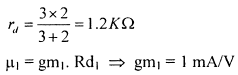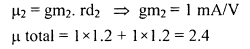QUESTION: 8

Which of the following semi-conductors are used for solar-cells?

1. Si     2. Ge    3. GaAs     4. CdS

Solution: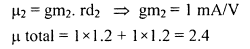QUESTION: 9

Temp. Coefficient of resistivity in an Intrinsic Semiconductor is

Solution:
QUESTION: 10

Under low level injection assumption, the main driving current in an extrinsic semiconductor of n-type is

Solution:

Under low level injection, hold drift current being directly proportional to concentration of holes is negligible.

QUESTION: 11

The following statements are related to a tunnel diode

1. Fermi level in n-side lies inside valence band

2. Excellent conduction is possible in reverse bias

3. In reverse bias negative resistance is exhibited

4. VP > VV

5. It can act as oscillator

6. The magnitude of lP is decided by impurity concentration and Junction area

7. Ge tunnel diode is used for commercial applications

Which of the above statements are false?

Solution:
QUESTION: 12

For a NPN transistor which operates in active region then main stream of current in base is

Solution:
QUESTION: 13

Which of the following diodes work under reverse bias condition? 1. Zener 2. Varacter 3. Photo diode 4. LED

Solution:

LED works in forward biased condition.

QUESTION: 14

In a forward bias photodiode an increase in incident light intensity causes diode current to

Solution:

Due to light new electrons and hole pairs are produced.

QUESTION: 15

In an unbiased P-N Junction zero current implies that

Solution:Use Code STAYHOME200 and get INR 200 additional OFF Use Coupon Code